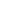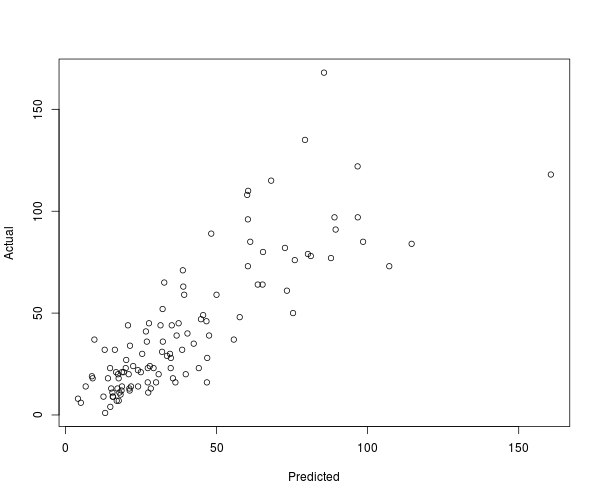CRAN package by Andrie de Vries

• and many others

R interface to AzureML and the AzureML Studio

• List, consume, and publish AzureML web services

Would not have been possible with out the Python package by Dino Viehland

Thanks!

Best audience (IMO):

Azure users who want to add R to their mix

## Check it out

library(AzureML)
ws <- workspace()

m <- glm(Ozone ~ ., data=airquality[sample(nrow(airquality), 100),], family=poisson)

fun <- function(newdata) predict(m, newdata=newdata, type="response")

ep <- publishWebService(ws, fun=fun, name="Ozone",
inputSchema=airquality,
data.frame=TRUE)

ans <- consume(ep, airquality)$ans plot(ans, airquality$Ozone)
library(AzureML)
ws <- workspace()

m <- glm(Ozone ~ ., data=airquality[sample(nrow(airquality), 100),], family=poisson)

fun <- function(newdata) predict(m, newdata=newdata, type="response")

ep <- publishWebService(ws, fun=fun, name="Ozone",
inputSchema=airquality,
data.frame=TRUE)

ans <- consume(ep, airquality)$ans plot(ans, airquality$Ozone)Connect to the AzureML Studio service

library(AzureML)
ws <- workspace()

m <- glm(Ozone ~ ., data=airquality[sample(nrow(airquality), 100),], family=poisson)

fun <- function(newdata) predict(m, newdata=newdata, type="response")

ep <- publishWebService(ws, fun=fun, name="Ozone",
inputSchema=airquality,
data.frame=TRUE)

ans <- consume(ep, airquality)$ans plot(ans, airquality$Ozone)Train a really basic model

library(AzureML)
ws <- workspace()

m <- glm(Ozone ~ ., data=airquality[sample(nrow(airquality), 100),], family=poisson)

fun <- function(newdata) predict(m, newdata=newdata, type="response")

ep <- publishWebService(ws, fun=fun, name="Ozone",
inputSchema=airquality,
data.frame=TRUE)

ans <- consume(ep, airquality)$ans plot(ans, airquality$Ozone)Define a prediction function based on the model.
Note the scoping of m.

library(AzureML)
ws <- workspace()

m <- glm(Ozone ~ ., data=airquality[sample(nrow(airquality), 100),], family=poisson)

fun <- function(newdata) predict(m, newdata=newdata, type="response")

ep <- publishWebService(ws, fun=fun, name="Ozone",
inputSchema=airquality,
data.frame=TRUE)

ans <- consume(ep, airquality)$ans plot(ans, airquality$Ozone)Publish the prediction function as a web service.
Lexical scope works!

library(AzureML)
ws <- workspace()

m <- glm(Ozone ~ ., data=airquality[sample(nrow(airquality), 100),], family=poisson)

fun <- function(newdata) predict(m, newdata=newdata, type="response")

ep <- publishWebService(ws, fun=fun, name="Ozone",
inputSchema=airquality,
data.frame=TRUE)

ans <- consume(ep, airquality)$ans plot(ans, airquality$Ozone)Call the service, using the whole data set as data for prediction.Hacker service!

v <- publishWebService(ws,
fun =  function(expr)
paste(capture.output(eval(parse(text=expr))), collapse = "\n"),
name="commander",
inputSchema = list(x = "character"), outputSchema=list(foo = "character"))

cat(consume(v, list(x = "Sys.info()"))\$foo)

#        sysname         release         version        nodename         machine
#      "Windows"         "7 x64"    "build 9200"        "CLIENT"        "x86-64" 

## What next?

API interface is under revision

(mostly under the hood stuff)

Things to improve:

• Data marshling is TSV/CSV right now, kinda sucks :(
• Use the .NET/mono array class?
• Use Apache Arrow/feather? [yes, use this]    :-)
• Ditto for Python
• Maybe just drop the whole outputSchema thing?
• I want an interface to the blob storage service
• Integration with R parallel/distributed programming tools
• foreach (from Revolution!)
• Ditto for Python (celery? or maybe that Jupyter 0MQ thing?)
• Need a formal AzureML studio API specification! >:(